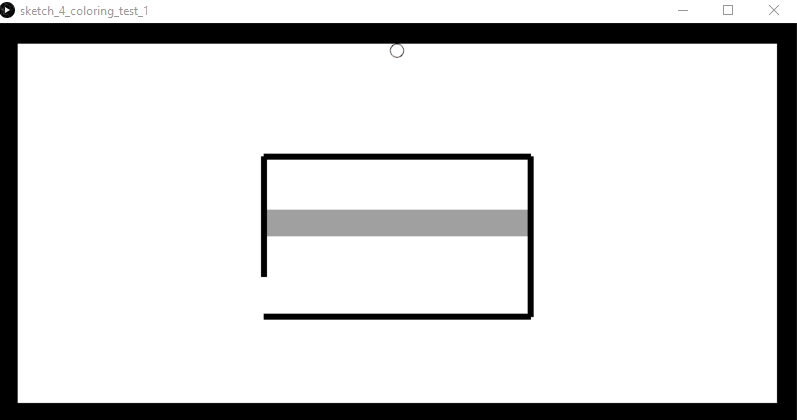# Setting Up First Sketching Prototype

`if (true) {    angle += 5;    float val = cos(radians(angle)) * 12.0;    for (int a = 0; a < 360; a += 75) {      float xoff = cos(radians(a)) * val;      float yoff = sin(radians(a)) * val;      fill(0);      ellipse((corr_posEE.x)*40 + xoff, (corr_posEE.y)*40 + yoff,      val, val);    }    fill(255);    ellipse((corr_posEE.x)*40, (corr_posEE.y)*40, 2, 2);  }`the tool shows the overlaid ‘tracing’ element but leaving no traces (code)
`void draw() {  /* put graphical code here, runs repeatedly at defined framerate in setup, else default at 60fps: */  if (renderingForce == false) {    background(255);    world.draw();  }`
`public void b1(int theValue) {  tooltip = button_img;  haplyAvatar = loadImage(tooltip);   haplyAvatar.resize((int)(hAPI_Fisica.worldToScreen(tooltipsize)), (int)(hAPI_Fisica.worldToScreen(tooltipsize)));  playerToken.h_avatar.attachImage(haplyAvatar);}`